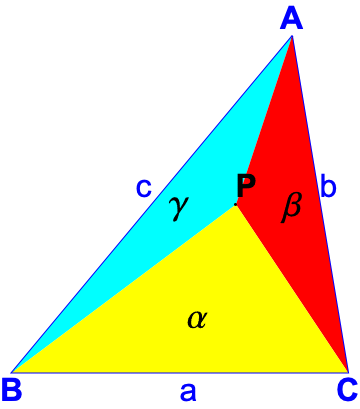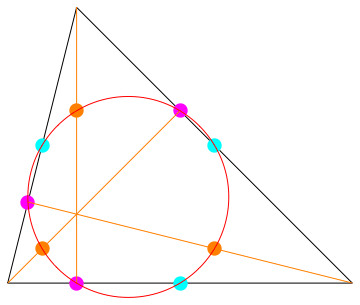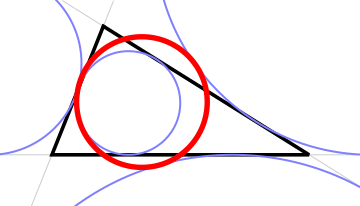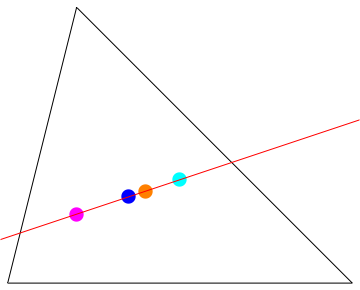## Triangle Convertor for Cartesian, Trilinear and Barycentric Coordinates

### Names and Terms

The triangle has vertices A, B and C and these names also refer to the triangle's angles at these vertices.
The side opposite vertex A is BC and is also called a which is its length; similarly for sides b and c.
Cartesian Coordinates
the (x,y) coordinates of the three vertices A, B and C
Trilinear CoordinatesThe 3 distances of a point from each of the sides of the triangle. If point P is (signed) distance Ta from side BC, Tb from side AC and Tc from side AB then its exact trilinear coordinates are written as Ta:Tb:Tc. A point on the same side of BC as vertex A has positive distance, 0 if on the line and a negative distance if on the other side of that line; similarly for the other sides. So in the diagram here, Ta and Tb are positive and Tc is negative. Ta:Tb:Tc is the same point as k×Ta : k×Tb : k×Tc for any non-zero number k. These are called Normalised trilinear coordinates. For example 1:1:1 is the point an equal distance from all three sides so these are the normalised trilinear coordinates of the centre of the inscribed circle of the triangle, or the incenter 1:0:0 is the vertex A, 0:1:0 is the vertex B, 0:0:1 is the vertex C If A⊥BC means the altitude of vertex A from side BC then: A has trilinears A⊥BC:0:0, B is 0:B⊥AC:0, C is 0:0:C⊥AB.
Barycentric Coordinates (also known as the areal or area coordinates)A point P is represented by the signed areas of the three sub-triangles made by joining P to vertices A, B and C, called the exact barycentric coordinates and written as {area of PBC, area of PAC, area of PBA} often written as {α, β, γ} . The sum of the exact barycentric coordinates, namely α+β+γ must be the area of the triangle ABC. Normalised barycentric coordinates are some (non-zero) multiple of the exact areas If a point has exact trilinear coordinates Ta : Tb : Tc then its exact barycentric coordinates are {a Ta/2, b Tb/2, c Tc/2} which can be normalised to {a Ta, b Tb, c Tc} If a point has exact barycentric coordinates {α, β, γ} then its exact trilinear coordinates are 2 α/a : 2 β/b : 2 γ/c which can be normalised to α/a : β/b : γ/c If P is outside the triangle, then some areas are negative. The sign of the area is the same as for trilinear coordinates.
Points at infinity
The sum of signed exact barycentric coordinates is defined to be the area of the circle. Barycentric coordinates that sum to 0 can still be useful. They do not represent points with finite cartesian coordinates and so are called points at infinity or, since there are many different barycentric triples with sum 0, they are also called points on the 'line' at infinity.
Similarly, there are points whose trilinears when converted to barycentrics sum to 0.
The normalized barycentric coordinates for these points have a maximum absolute coordinate value equal to 1.
The ETC centers X(30) and X(511) through to X(519) are examples.
Nine-point circleThe following 9 points all lie on the same circle:
• The foot of each altitude the point on a side where a line perpendicular to that side goes through the other vertex
Trilinears 0:cos(C):cos(B), cos(C):0:cos(A), cos(B):cos(A):0
• the mid-points of the sides
Trilinears: 0:1:1, 1:0:1, 1:1:0
• the mid-point of the lines from the orthocentre to the vertices

Its radius is one half of the circumcircle through all 3 vertices.
Feuerbach's Theorem of 1822 states that the nine-point circle is tangent to the incircle and the three excircles.

The Euler lineThe following points are on the same line:
• the centroid where the lines joining a vertex to the mid-point of the side opposite all meet
Barycentrics {1,1,1}
• the circumcentre of the circle through all 3 vertices
Trilinears cos(A):cos(B):cos(C)
• the orthocentre where the three altitudes meet
Barycentrics {tan(A),tan(B),tan(C)}
• the 9-point centre Trilinears cos(B-C):cos(C-A):cos(A-B)

Many other ETC centers lie on the Euler line. A list is given on Eric W Weisstein's "Euler Line" Mathworld page - see Links and References at the foot of this page.

### Triangle coordinates converter

#### How to use the Coordinate Conversion Calculator

• Enter the triangle's vertices as
• the 6 cartesian coordinates of the 3 vertices in the 6 input boxes
• or the 3 side lengths and then click on the button to have the computed the coordinates put into the 6 coordinates boxes
• Optionally press the button to get the triangle's data shown in the RESULTS:
side lengths, area, angles, altitudes and other values used in the ETC.
They are shown to the accuracy specified in the Number of Decimal Places box
• Enter either a point P's cartesian coordinates or trilinear coordinates or its barycentric values, normalised or exact.
• Press the conversion button under the coordinates you entered.
• The results are printed in the RESULTS to the accuracy you specified in the Number of Decimal Places box
The side-lengths, area and altitudes are calculated each time a coordinate's conversion button is pressed using the triangle's vertex ccoordinates.

#### Expressions or Numbers may be entered for any value

Show Help on Expression input
Number of decimal places
You can control the precision (number of decimal places) of all results by altering the value in the Working Precision input box. Altering the value and then pressing the appropriate Calculation button will recompute values to the new precision.
Numbers
• Must begin with a digit or a sign. A decimal point (.) is optional. Spaces are ignored.
• For scientific notation use e or E to mean times 10 to the power of which must be preceded AND followed by an optionally signed integer
for example 1 000 = +0.1e4 =1E3 = 10000E-1 are all valid forms for a decimal number.
• For numbers in bases other than 10, from 2 to 35, enclose the digits in single quotes (') and follow the second quote by the base, for example '101'2 is the binary value 1012 = 5.
Digits after 9 in bases 11 to 35 are the letters a to z or A to Z as the case does not matter. For example, 'a'12 is a12 which is 10 in base 10 and 'z'36 is 3510
• Numbers in other bases can include a 'decimal' point. For example '1010.101'2 = 10.625 = 85/8 = 10+5/8
• To convert a base 10 number to another base, use the tobase function - see below.
Arithmetic operators
Use +, -, / as usual but use * for all multiplications. + and - may be unary operators as in -(2+3)
^ means to the power of
Note that 2^3 means 2 to the power of 3 = 2*2*2=8 whereas 2e3 means 2 times 10 to the power of 3 = 2*10^3 = 2000.
Rules a^(-b) = 1/a^b; a^(b+c) = a^b*a^c. Examples:
// (quotient) and % (remainder)
// means "integer divide" so that a//b means the integer part of a/b or the whole number quotient.
The remainder when a is divided by b is a%b = mod(a,b)
Theorems
Since a/b = quotient + remainder/b then we always have
Theorems (Rules): (a−a%b)/b = a//b = trunc(a/b); a//b*b+a%b = a
For example: 21/5 is 4 and 1/5 so the quotient 21//5 is 4 and the remainder 21%5 is 1.
Negative a or b
The quotient a//b is negative if and only if only one of a and b is negative; the remainder always has the same sign as a.
This assures that the Rules above are always true for all values of a (the dividend) and b (the divisor).
Priority of operators
Operator priority (binding power):
 Highest . . . . . . . . . . Lowest unary + unary − ^ */%// binary +binary −
If @ and Δ are two operators then
@ binds more tightly than Δ means the same as
@ has a higher priority than Δ
so that 2 @ 1 Δ 3 means (2 @ 1) Δ 3.
Brackets are needed for the expression: 2 @ (1 Δ 3).
If on the other hand Δ had a higher priority than @ then 2 @ 1 Δ 3 would mean 2 @ (1 Δ 3) and brackets would be needed for (2 @ 1 ) Δ 3.
Equal priority operators act from the left (they are left-associative) so that 2-3-4 means (2-3)-4 = -5
2^3^3 = (2^3)^3 = 8^3 = 512
2*4%5 = (2*4)%5 = 3
For example -2^4 means (-2)^4 = 16; use -(2^4) otherwise
2^1/2 mean (2^1)/2; use 2^(1/2) or 2^0.5 or sqrt(2) for the square-root of 2.
Constants
The only built-in constants are:
• Pi or pi = 3.14159... . This is computed as 4*arctan(1) to the Working precision.
• E or e = 2.71828... : E = [2; 1,2,1, 1,4,1, 1,6,1, 1,8,1, 1,10,1, ...]
• Phi and phi the golden ratios, Phi = (sqrt(5) + 1)/2 = 1.618... and phi = (sqrt(5) − 1)/2 = 0.618..
These values are connected and defined by Phi = 1/phi = phi+1; phi = 1/Phi = Phi − 1
Phi = [1; 1 ];
Apart from Phi and phi, constants can be in UPPERCASE or lowercase.
Functions
a name (using UPPER or lower case letters) followed by arguments in brackets separated by a comma: for example sqrt(2.3) and log(2,10)
• tobase(n,b) convert (base 10 number) n to base b (in range 2 to 36). for example tobase(14,2) is 1110
• trunc(x) truncate x: forget any digits after the decimal point but retain the sign
trunc(2.9) is 2; trunc(-2.3) = -2 = trunc(-2.9); trunc(2.3) = 2
• round(x) rounds x to the nearest integer
round(2.9) = 3; round(-2.3) = -2; round(-2.9) = -3; round(2.3) = 2;
• abs(x) the absolute value of x: x if x≥0 and -x if x<0
• sqrt(x) the square-root of x: for example sqrt(2) = 1.414... is the same as 2^0.5
• mod(x,d) the remainder when x is divided by d. The remainder has the same sign as x
• powmod(x,p,d) the remainder when xp is divided by d. This should be used for large x, p or d to avoid loss of precision calculating xp when this value is very large. It is equivalent to mod(x^p,d) but is much faster.
Trigonometric functions:
• sin(x) the sine of an angle x in radians
• cosec(x) the cosecant of an angle x in radians = 1/sin(angle)
• cos(x) the cosine of an angle x in radians
• sec(x) the secant of an angle x in radians = 1/cos(angle)
• tan(x) the tangent of an angle x in radians
• cot(x) the cotangent of an angle x in radians = 1/tan(angle)
• arcsin(s) gives the angle in radians whose sine is s. The angle returned is between -PI/2 and PI/2 (±1.57079632...) radians.
• arccos(c) gives the angle in radians whose cosine is c. The angle returned is between 0 and PI radians.
• arctan(t) gives the angle in radians whose tangent is t. The angle returned is between -PI/2 and PI/2 (±1.57079632...) radians.
Functions related to e:
• log(x) which is the natural log of x: for example log(E^2) is 2
• log(b, x) finds log to base b of x: log to base 2 of 8 (=23) is log(2,2^3) = 3
How many digits has fib(200)? log(10,fib(200)) = 41.44.. to it has 45 digits
• exp(x) which is E to the power of x; for example exp(3.4) is the same as E^3.4
exp(1/2) = E^(1/2) = sqrt(E) = [1; 1, 1,1,5, 1,1,9, 1,1,13, 1,1,17, 1,1,21, ...]
exp(1/3) = E^(1/3) = [1; 2, 1,1,8, 1,1,14, 1,1,20, 1,1,26, 1,1,32, ...]
• sinh(x) the hyperbolic sine defined as (Ex−E-x)/2
• cosh(x) the hyperbolic cosine defined as (Ex+E-x)/2 which will be ≥1
• tanh(x) the hyperbolic tangent defined as sinh(x)/cosh(x). -1≤tanh(x)≤1 for all x
tanh(1) as a CF is [0;1, 3, 5, 7, 9, 11, 13, ...]
tanh(1/2) as a CF is [0;2, 6, 10, 14, 18, 22,...]
• arcsinh(x) the inverse hyperbolic sine defined as the value y for which sinh(y)=x
• arccosh(x) the inverse hyperbolic cosine defined as the value y for which cosh(y)=x
only defined if x≥1 and returns NaN otherwise
• arctanh(x) the inverse hyperbolic tangent defined as the value y for which tanh(y)=x
only defined if −1≤x≤1
Other functions
• champ(b), the Champernowne number in base b: see The decimal number 0.12345... .
The argument is the base of the numbers. champ(10)=0.1234567891011121314... . Bases are 2 to 10 and the resulting sequence (the numbers 0,1,2,... but in base b) is converted to a decimal number. For example: champ(2) is 0.1 10 11 100 101 ... in base 2 which is 0.8622401258680545... as a decimal number. To see the base 2 form, use tobase(champ(2),2)
See Champernowne numbers in different bases
• fib(n) The n-th Fibonacci number where
fib(0)=0, fib(1)=1 and fib(n)=fib(n-1)+fib(n-2). n is an integer and can be negative.
RULE: fib(n) = (Phi^n-(-phi)^n)/sqrt(5)
• luc(n) the n-th Lucas number where luc(0)=2, luc(1)=1, luc(n)=luc(n-1)+luc(n-2). n is an integer and can be negative.
RULE: luc(n) = Phi^n + (-phi)^n
• gfib(a,b,n) the n-th General Fibonacci number where
gfib(a,b,0)=a, gfib(a,b,1)=b, gfib(a,b,n)=gfib(a,b,n-1)+gfib(a,b,n-2). n is an integer and can be negative.
RULE: gfib(a,b,n) = a*fib(n-1) + b*fib(n)
• primefactors(n) find the prime factors of n
Will find all the prime factors of n that are less than 1013 (otherwise testing a prime larger than this would take more than 10 seconds or so). Any unresolved divisors will have no prime factors below this limit and will be shown like this. The result is shown as primepower×primepower...
primefactors(31622816796611496307) = 31622772×3162283
primefactors(10000021122911) = 10000021122911 is prime
primefactors(10000021122989) = 10000021122989 has no prime factors <1013

#### The Coordinate Converter

You may use the names a,b,c for the triangle's sides, A,B,C for its angles and area in the coordinate input boxes.
The button will copy a formula (expresssion) from the top input box to the two input boxes below it, rotating the sides a,b,c and angles A,B,C each time.
When inputting a list of coordinates or a list of the sides, a SPACE separates the values.
Set the Show numbers to decimal places to the accuracy required for all values displayed in RESULTS, up to 100 decimal places.

• To see if a point is a known ETC center:
• Make sure the sides 6 9 13 are used and transferred to the Triangle's vertices inputs.
• Input Cartesian, Trilinear or Barycentric coordinates for a point relative to the 6 9 13 triangle.
• If the point corresponds to a known center in the ETC, a link to that center will be shown in the RESULTS
• To find the ETC information on a particular X Center:
• Fill in the X center number and then use the to get a link to the ETC center in the RESULTS box.
• Clicking on the link produced will open a separate page/tab in your browser showing the ETC entry for that center.
• All ETC links will open in that same page/tab each time.
• This version uses the ETC tables updated on

C A L C U L A T O R
Use 6 9 13 to search for ETC centers
sides:
 The triangle's vertices: A: (  ,  ) B: (  ,  ) C: (  ,  )
Select Coordinates to convert:
to ETC Center X()

R E S U L T S      Show numbers to decimal places

 © 2019 Dr Ron KnottBack to Dr Knott's Maths HOME page